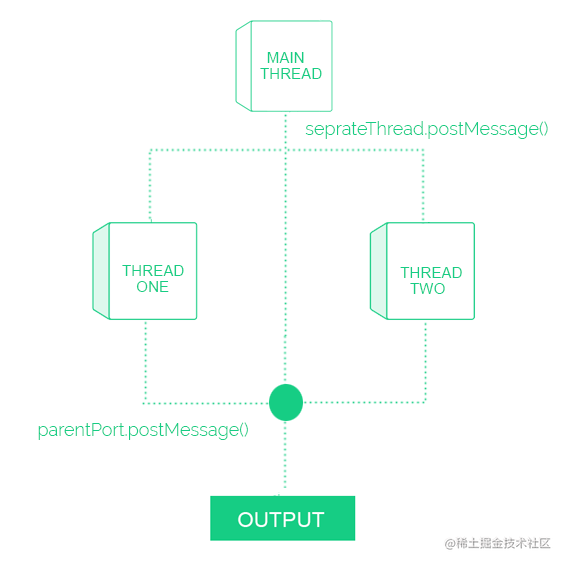### 译|如何使用 Node.js 执行多线程从 Node.js 的第 13 版开始，它就能够执行多线程。

## 实现

``````/*
*  文件名：index.js
*  描述：这是一个主线程
*/

const express = require("express");

const app = express();
const port = 3000;

app.get("/", (req, res) => {
});

});

app.listen(port, () => {
console.log(`Example app listening at http://localhost:\${port}`);
});

``````/*
*  文件名：index.js
*  描述：这是一个主线程
*/

const express = require("express");

const app = express();
const port = 3000;

const getSum = (limit) => {
let sum = 0;
for (let i = 0; i < limit; i++) {
sum += i;
}
return sum;
};

app.get("/", (req, res) => {

const result = getSum(1000);
res.send(`Processed function getSum on main thread and result: \${result}`);

});

res.send("Process function getSum on seprate thread.");
});

app.listen(port, () => {
console.log(`Example app listening at http://localhost:\${port}`);
});

• 将工作线程模块导入到主线程。
``````const { Worker } = require("worker_threads");

• 创建工作线程模块的实例，并提供新创建文件的路径名。
``````const seprateThread = new Worker(__dirname + "/seprateThread.js");

• 启动新线程。
``````seprateThread.on("message", (result) => {
res.send(`Processed function getSum on seprate thread:  \${result}`);
});

• 将数据发送到新线程。
``````seprateThread.postMessage(1000);

``````/*
*  文件名：index.js
*  描述：这是一个主线程
*/

const express = require("express");
const { Worker } = require("worker_threads");

const app = express();
const port = 3000;

const getSum = (limit) => {
let sum = 0;
for (let i = 0; i < limit; i++) {
sum += i;
}
return sum;
};

app.get("/", (req, res) => {

const result = getSum(1000);
res.send(`Processed function getSum on main thread and result: \${result}`);

});

res.send(`Processed function getSum on seprate thread: \${result}`);
});

});

app.listen(port, () => {
console.log(`Example app listening at http://localhost:\${port}`);
});

``````/*
*  描述：这是一个另一个线程
*/

const { parentPort } = require("worker_threads");

const getSum = (limit) => {
let sum = 0;
for (let i = 0; i < limit; i++) {
sum += i;
}
return sum;
};

parentPort.on("message", (limit) => {
const result = getSum(limit);
parentPort.postMessage(result);
});## 特点

• 每个线程都有单独的 V8 引擎。
• 子线程可以相互通信。
• 子线程可以共享相同的内存。
• 初始值可以在启动新线程时作为选项传递。

## 结论486